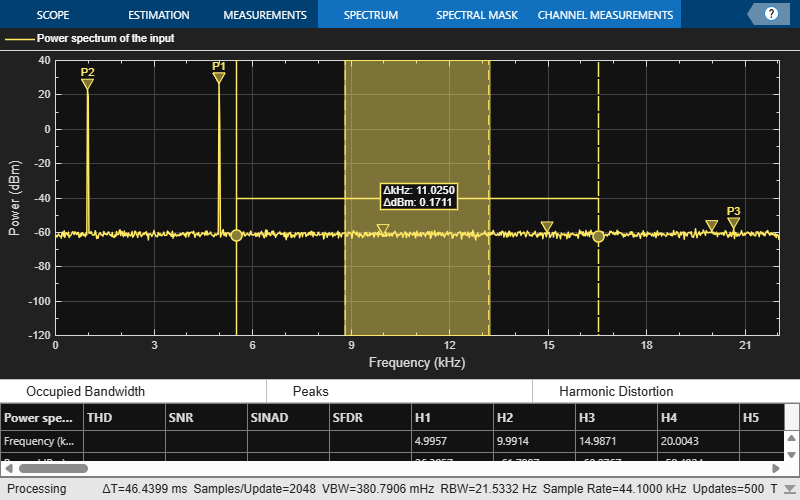# Obtain Measurements Data Programmatically for `spectrumAnalyzer` object

Compute and display the power spectrum of a noisy sinusoidal input signal using the `spectrumAnalyzer` MATLAB® object. Measure the peaks, cursor placements, adjacent channel power ratio, and distortion values in the spectrum by enabling these properties:

• `PeakFinder`

• `CursorMeasurements`

• `ChannelMeasurements`

• `DistortionMeasurements`

### Initialization

The input sine wave has two frequencies: 1000 Hz and 5000 Hz. Create two `dsp.SineWave` System objects to generate these two frequencies. Create a `spectrumAnalyzer` object to compute and display the power spectrum.

```Fs = 44100; Sineobject1 = dsp.SineWave(SamplesPerFrame=1024,PhaseOffset=10,... SampleRate=Fs,Frequency=1000); Sineobject2 = dsp.SineWave(SamplesPerFrame=1024,... SampleRate=Fs,Frequency=5000); SA = spectrumAnalyzer(SampleRate=Fs,SpectrumType="power",... PlotAsTwoSidedSpectrum=false,ChannelNames={'Power spectrum of the input'},... YLimits=[-120 40],ShowLegend=true);```

### Enable Measurements Data

To obtain the measurements, set the `Enabled` property to `true`.

```SA.CursorMeasurements.Enabled = true; SA.ChannelMeasurements.Enabled = true; SA.PeakFinder.Enabled = true; SA.DistortionMeasurements.Enabled = true;```

### Use `getMeasurementsData`

Stream in the noisy sine wave input signal and estimate the power spectrum of the signal using the `spectrumAnalyzer` object. Measure the characteristics of the spectrum. Use the `getMeasurementsData` function to obtain these measurements programmatically. The `isNewDataReady` function returns `true` when there is new spectrum data. Store the measured data in the variable `data`.

```data = []; for Iter = 1:1000 Sinewave1 = Sineobject1(); Sinewave2 = Sineobject2(); Input = Sinewave1 + Sinewave2; NoisyInput = Input + 0.001*randn(1024,1); SA(NoisyInput); if SA.isNewDataReady data = [data;getMeasurementsData(SA)]; end end```The panes at the bottom of the scope window display the measurements that you have enabled. The values in these panes match the values in the last time step of the `data` variable. You can access the individual fields of `data` to obtain the various measurements programmatically.

### Compare Peak Values

Use the `PeakFinder` property to obtain peak values. Verify that the peak values in the last time step of `data` match the values in the spectrum analyzer plot.

`peakvalues = data.PeakFinder(end).Value `
```peakvalues = 3×1 26.3957 22.7830 -57.9977 ```
`frequencieskHz = data.PeakFinder(end).Frequency/1000`
```frequencieskHz = 3×1 4.9957 0.9905 20.6719 ```